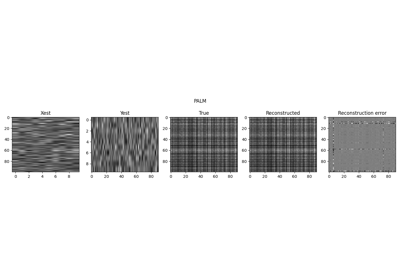# pyproximal.utils.bilinear.LowRankFactorizedMatrix#

class pyproximal.utils.bilinear.LowRankFactorizedMatrix(X, Y, d, Op=None)[source]#

Low-Rank Factorized Matrix operator.

Bilinear operator representing the L2 norm of a Low-Rank Factorized Matrix defined as: $$H(\mathbf{X}, \mathbf{Y}) = \frac{1}{2} \|\mathbf{Op}(\mathbf{X}\mathbf{Y}) - \mathbf{d}\|_2^2$$, where $$\mathbf{X}$$ is a matrix of size $$n \times k$$, $$\mathbf{Y}$$ is a matrix of size $$k \times m$$, and $$\mathbf{Op}$$ is a linear operator of size $$p \times n$$.

Parameters
Xnumpy.ndarray

Left-matrix of size $$n \times k$$

Ynumpy.ndarray

Right-matrix of size $$k \times m$$

dnumpy.ndarray

Data vector

Oppylops.LinearOperator, optional

Linear operator

Notes

The Low-Rank Factorized Matrix operator has gradient with respect to x equal to:

$\nabla_x H(\mathbf{x};\ mathbf{y}) = \mathbf{Op}^H(\mathbf{Op}(\mathbf{X}\mathbf{Y}) - \mathbf{d})\mathbf{Y}^H$

and gradient with respect to y equal to:

$\nabla_y H(\mathbf{y}; \mathbf{x}) = \mathbf{X}^H \mathbf{Op}^H(\mathbf{Op} (\mathbf{X}\mathbf{Y}) - \mathbf{d})$

Note that in both cases, the currently stored x/y is used for the second variable within parenthesis (after ;)

Methods

 __init__(X, Y, d[, Op]) grad(x) gradx(x) grady(y) lx(x) ly(y) matvec(x) updatex(x) Update x variable (to be used to update the internal variable x) updatexy(x) updatey(y) Update y variable (to be used to update the internal variable y)

## Examples using pyproximal.utils.bilinear.LowRankFactorizedMatrix#Low-Rank completion via Matrix factorization

Low-Rank completion via Matrix factorization